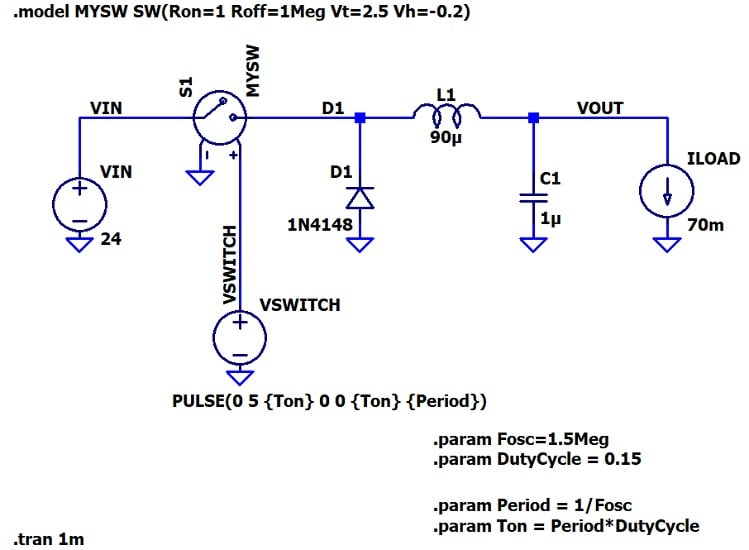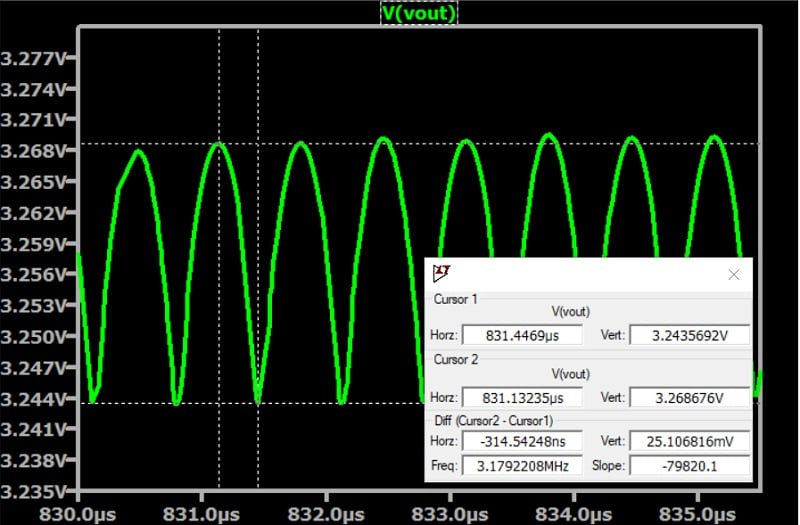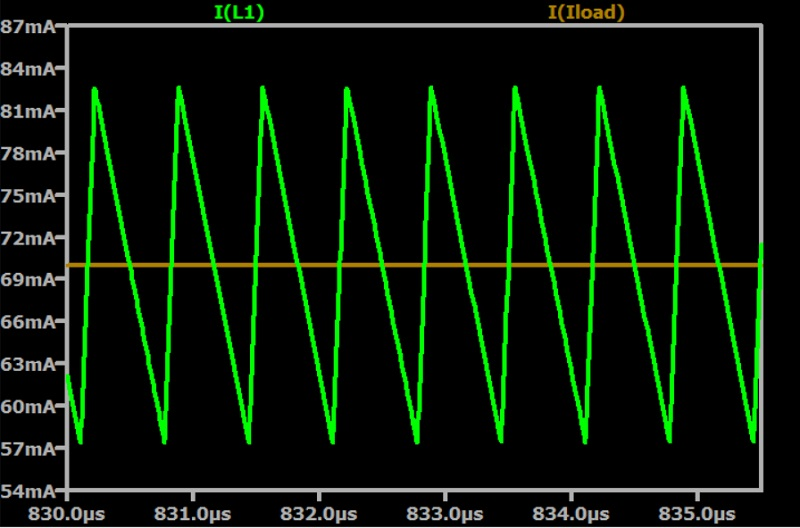Technical Article

# Choosing Capacitance Values for a Step-Down Switching Regulator

August 30, 2023 by Robert Keim

## Designing a buck converter? Read this article for practical guidance on selecting an output capacitance value.

In my last article, we discussed key characteristics of ceramic, aluminum electrolytic, and tantalum capacitors; we then investigated the role of output-capacitor equivalent series resistance (ESR) in the design of switch-mode regulators. Now we’ll consider the trade-offs involved in selecting a capacitance value and work through a design example based on desired output ripple. All LTspice simulations will use the schematic in Figure 1, though the value of C1 will change.##### Figure 1. An LTspice schematic of a buck converter.

Higher and lower capacitance values both have their own pros and cons: you’ll need to decide what value best balances them for your intended application. To that end, let’s look briefly at both sets of advantages.

#### Advantages of Lower Output Capacitance

As we touched on in the preceding article, capacitor type affects capacitance value. In a sense, capacitance value also helps determine capacitor type: for example, choosing a high output capacitance all but rules out using a ceramic capacitor. Since ceramic capacitors offer low ESR and are generally preferable for SMPS circuits, it may be worth choosing a lower capacitance value to take advantage of this technology.

Smaller ceramic-capacitor packages have lower inductance and are consequently more effective at high frequencies; additionally, a requirement for lower output capacitance typically translates to a less expensive, less bulky capacitor.

Finally, keeping output capacitance low avoids the problem of excessive output capacitance, which can cause the regulator to draw so much current at startup that it enters an overcurrent-protection mode.

#### Advantages of Higher Output Capacitance

With electrolytic capacitors, ESR tends to decrease as capacitance increases. Also (and regardless of capacitor type), output ripple magnitude is inversely proportional to output capacitance: more COUT means less ΔVOUT.

Keep in mind that output ripple is a major consideration. Ripply output voltage is one of the fundamental weaknesses of switch-mode power supplies relative to linear regulators. In some applications, minimizing output ripple is an important design objective.

### Calculating Capacitance According to Output Ripple

If you have a maximum output ripple specification in mind, you can use that as a basis for choosing a capacitance value. Here’s the formula:

$$C_{OUT}=\frac{\Delta I_L}{8\times f_S\times\Delta V_{OUT}}$$

Let’s imagine that we’re using this formula to choose an initial value for COUT, and that we don’t yet have a simulated value for inductor current ripple (ΔIL). In this case, we can use the 30% rule that we first saw in the article on choosing an inductance value: we aim for peak-to-peak inductor current ripple that is 30% of expected output current, i.e., ΔIL = 0.30 × 70 mA = 21 mA.

We’ve already chosen a switching frequency (fS) of 1.5 MHz. Now we just need to choose a spec for ΔVOUT.

Though the formula above implies that you can just keep adding output capacitance until the ripple disappears, in real life there are practical limits. As an example, the LTM4702 from Analog Devices is part of the “Silent Switcher” line of low-noise switching regulators. The people who designed this component know vastly more about optimizing switcher performance than I ever will, and yet the LTM4702’s typical output ripple is 8 mV (and that’s with 200 μF of output capacitance).

Fortunately, while it’s difficult to know how low the ripple really needs to be for a given application, in my experience low-voltage circuitry—even analog circuitry—is quite resistant to noise on the power line (let’s not forget that every IC already has its own bypass capacitor).

I think that ΔVOUT = 20 mV is an achievable goal, so we’ll use that as our starting point. If we run the numbers at ΔVOUT = 20 mV, we obtain the following:

$$C_{OUT}=\frac{.021\ A}{8\times 1500000\ Hz\times .020\ V}=87.5\ nF$$

Now let’s go back to the LTspice schematic and change the value of C1 to 87.5 nF. Figure 2 shows a plot of VOUT:##### Figure 2. Simulated voltage output of the buck converter in Figure 1 with C1 set to 87.5 nF.

As you can see in the cursor box, the peak-to-peak variation in VOUT is quite close to our 20 mV theoretical value.

We can also check the inductor current to see if this new capacitor value—which is significantly smaller than the 1 μF default value that we started with—has caused any undesirable changes. As the next plot (Figure 3) shows, our ΔIL is in the vicinity of 21 mA, with peaks extending about 13 mA above and 13 mA below the 70 mA load current.### Calculating Capacitance According to Overshoot

In some applications, there is no great need to reduce output ripple. A purely digital circuit, for example, might operate normally and reliably even with much greater than 20 mV of ripple on the power-supply voltage.

These applications can, however, be subject to abrupt changes in load current. Because the inductor in a switch-mode regulator has limited ability to react to such changes, the output capacitor plays an important role in supplying load current during transient events; it therefore makes sense to select the output capacitor based on transient response rather than ΔVOUT in these cases.

A load-current transient event will cause a temporary VOUT deviation, which is denoted by VOS in the formula below (“OS” stands for overshoot, but the deviation can also be undershoot). If you know your maximum expected change in load current and have a VOS target in mind, you can use the following formula to calculate an initial value for COUT:

$$C_{OUT}=\frac{(\Delta I_{OUT})^2 \times L}{2\times V_{OUT} \times V_{OS}}$$

As an aside, all capacitance-calculation formulas presented in this article can be found in this application note from Texas Instruments.

### Wrapping Up

With that, we’ve looked at two ways to determine an appropriate output capacitance for a buck converter and outlined the advantages of higher and lower capacitance values.

This article, together with the preceding one, covered capacitor selection for step-down switching regulators; the two articles before that dealt with choosing an inductor. While I plan to explore other switch-mode converter topologies in the future, this concludes my series on component selection for buck converters.

All images used courtesy of Robert Keim